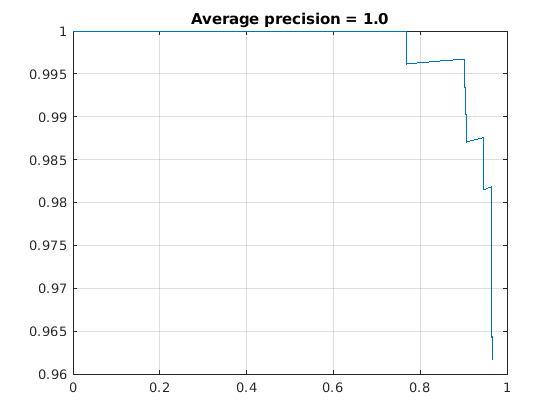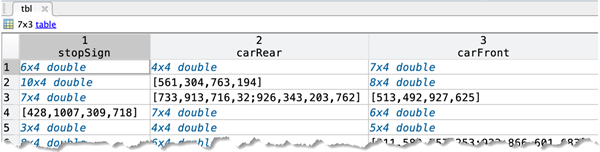Documentation

# evaluateDetectionPrecision

Evaluate precision metric for object detection

## Syntax

``averagePrecision = evaluateDetectionPrecision(detectionResults,groundTruthData)``
``````[averagePrecision,recall,precision] = evaluateDetectionPrecision(___)``````
``[___] = evaluateDetectionPrecision(___,threshold)``

## Description

````averagePrecision = evaluateDetectionPrecision(detectionResults,groundTruthData)` returns the average precision, of the `detectionResults` compared to the `groundTruthData`. You can use the average precision to measure the performance of an object detector. For a multiclass detector, the function returns `averagePrecision` as a vector of scores for each object class in the order specified by `groundTruthData`.```

example

``````[averagePrecision,recall,precision] = evaluateDetectionPrecision(___)``` returns data points for plotting the precision–recall curve, using input arguments from the previous syntax.```
````[___] = evaluateDetectionPrecision(___,threshold)` specifies the overlap threshold for assigning a detection to a ground truth box.```

## Examples

collapse all

This example shows how to evaluate a pretrained YOLO v2 object detector.

Load the Vehicle Ground Truth Data

Load a table containing the vehicle training data. The first column contains the training images, the remaining columns contain the labeled bounding boxes.

```data = load('vehicleTrainingData.mat'); trainingData = data.vehicleTrainingData;```

Add fullpath to the local vehicle data folder.

```dataDir = fullfile(toolboxdir('vision'), 'visiondata'); trainingData.imageFilename = fullfile(dataDir, trainingData.imageFilename);```

Create an imageDatastore using the files from the table.

`imds = imageDatastore(trainingData.imageFilename);`

Create a boxLabelDatastore using the label columns from the table.

`blds = boxLabelDatastore(trainingData(:,2:end));`

Load the detector containing the layerGraph for trainining.

```vehicleDetector = load('yolov2VehicleDetector.mat'); detector = vehicleDetector.detector;```

Evaluate and Plot the Results

Run the detector with imageDatastore.

`results = detect(detector, imds);`

Evaluate the results against the ground truth data.

`[ap, recall, precision] = evaluateDetectionPrecision(results, blds);`

Plot the precision/recall curve.

```figure; plot(recall, precision); grid on title(sprintf('Average precision = %.1f', ap))```Train an ACF-based detector using pre-loaded ground truth information. Run the detector on the training images. Evaluate the detector and display the precision-recall curve.

```load('stopSignsAndCars.mat') stopSigns = stopSignsAndCars(:,1:2); stopSigns.imageFilename = fullfile(toolboxdir('vision'),'visiondata', ... stopSigns.imageFilename);```

Train an ACF-based detector.

`detector = trainACFObjectDetector(stopSigns,'NegativeSamplesFactor',2);`
```ACF Object Detector Training The training will take 4 stages. The model size is 34x31. Sample positive examples(~100% Completed) Compute approximation coefficients...Completed. Compute aggregated channel features...Completed. -------------------------------------------- Stage 1: Sample negative examples(~100% Completed) Compute aggregated channel features...Completed. Train classifier with 42 positive examples and 84 negative examples...Completed. The trained classifier has 19 weak learners. -------------------------------------------- Stage 2: Sample negative examples(~100% Completed) Found 84 new negative examples for training. Compute aggregated channel features...Completed. Train classifier with 42 positive examples and 84 negative examples...Completed. The trained classifier has 20 weak learners. -------------------------------------------- Stage 3: Sample negative examples(~100% Completed) Found 84 new negative examples for training. Compute aggregated channel features...Completed. Train classifier with 42 positive examples and 84 negative examples...Completed. The trained classifier has 54 weak learners. -------------------------------------------- Stage 4: Sample negative examples(~100% Completed) Found 84 new negative examples for training. Compute aggregated channel features...Completed. Train classifier with 42 positive examples and 84 negative examples...Completed. The trained classifier has 61 weak learners. -------------------------------------------- ACF object detector training is completed. Elapsed time is 19.2586 seconds. ```

Create a table to store the results.

```numImages = height(stopSigns); results = table('Size',[numImages 2],... 'VariableTypes',{'cell','cell'},... 'VariableNames',{'Boxes','Scores'}); ```

Run the detector on the training images. Store the results as a table.

```for i = 1 : numImages I = imread(stopSigns.imageFilename{i}); [bboxes, scores] = detect(detector,I); results.Boxes{i} = bboxes; results.Scores{i} = scores; end ```

Evaluate the results against the ground truth data. Get the precision statistics.

`[ap,recall,precision] = evaluateDetectionPrecision(results,stopSigns(:,2));`

Plot the precision-recall curve.

```figure plot(recall,precision) grid on title(sprintf('Average Precision = %.1f',ap))```## Input Arguments

collapse all

Object locations and scores, specified as a two-column table containing the bounding boxes and scores for each detected object. For multiclass detection, a third column contains the predicted label for each detection. The bounding boxes must be stored in an M-by-4 cell array. The scores must be stored in an M-by-1 cell array, and the labels must be stored as a categorical vector.

When detecting objects, you can create the detection results table by using `imageDatastore`.

``` ds = imageDatastore(stopSigns.imageFilename); detectionResults = detect(detector,ds);```

Data Types: `table`

Labeled ground truth, specified as a datastore or a table.

Each bounding box must be in the format [x y width height].

• Datastore — A datastore whose `read` and `readall` functions return a cell array or a table with at least two columns of bounding box and labels cell vectors. The bounding boxes must be in a cell array of M-by-4 matrices in the format [x,y,width,height]. The datastore's `read` and `readall` functions must return one of the formats:

• Table — One or more columns. All columns contain bounding boxes. Each column must be a cell vector that contains M-by-4 matrices that represent a single object class, such as stopSign, carRear, or carFront . The columns contain 4-element double arrays of M bounding boxes in the format [x,y,width,height]. The format specifies the upper-left corner location and size of the bounding box in the corresponding image.Overlap threshold for assigned a detection to a ground truth box, specified as a numeric scalar. The overlap ratio is computed as the intersection over union.

## Output Arguments

collapse all

Average precision over all the detection results, returned as a numeric scalar or vector. Precision is a ratio of true positive instances to all positive instances of objects in the detector, based on the ground truth. For a multiclass detector, the average precision is a vector of average precision scores for each object class.

Recall values from each detection, returned as an M-by-1 vector of numeric scalars or as a cell array. The length of M equals 1 + the number of detections assigned to a class. For example, if your detection results contain 4 detections with class label `'car'`, then `recall` contains 5 elements. The first value of recall is always `0`.

Recall is a ratio of true positive instances to the sum of true positives and false negatives in the detector, based on the ground truth. For a multiclass detector, `recall` and `precision` are cell arrays, where each cell contains the data points for each object class.

Precision values from each detection, returned as an M-by-1 vector of numeric scalars or as a cell array. The length of M equals 1 + the number of detections assigned to a class. For example, if your detection results contain 4 detections with class label `'car'`, then `precision` contains 5 elements. The first value of `precision` is always `1`.

Precision is a ratio of true positive instances to all positive instances of objects in the detector, based on the ground truth. For a multi-class detector, `recall` and `precision` are cell arrays, where each cell contains the data points for each object class.